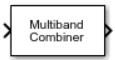# Multiband Combiner

Frequency-shift and combine signals

• Library:
• Communications Toolbox / RF Impairments and Components

•## Description

The Multiband Combiner block interpolates, shifts input signals to the specified frequency bands, and then combines them into a single signal. For more information, see the Algorithms section.

## Ports

### Input

expand all

Input signals, specified as an Nsamp-by-Nchan matrix. Nsamp is the number of input samples per channel and Nchan is the number of channels.

Data Types: `double` | `single`
Complex Number Support: Yes

### Output

expand all

Output signal, returned as an Nout-by-1 vector of the same data type as input signal `In`. Nout is the number of output samples. The Rate options parameter specifies single-rate or multirate mode. For more information, see Algorithms.

## Parameters

expand all

Input signal sample rate in Hz, specified as a positive scalar.

Frequency offsets in Hz, specified as one of these options.

• Scalar — Each channel of the input signal is frequency-shifted by this scalar value.

• 1-by-Nchan vector — Each channel of the input signal is frequency-shifted by the corresponding value in this vector. Nchan is the number of channels in the input signal `In`.

Options for the processing rate, specified as one of these values.

• `Enforce single-rate processing` — The output sample rate must be an integer multiple of the input sample rate. The number of rows in the output is higher than or equal to the number of rows in the input signal. The output frame rate is equal to the input frame rate.

• `Allow multirate processing` — The output has the same number of rows as the input. The output frame rate is higher than or equal to the input frame rate.

Options for the output sample rate, specified as one of these values.

• `Auto` — The block interpolates the input signals to ensure that the resulting sample rate of the signals is sufficient to avoid distorting the frequency content of the original signals after they are frequency-shifted to produce the output signal.

• `Specify via property` — Specify the output sample rate by using the Output sample rate (Hz) parameter.

Output signal sample rate in Hz, specified as a positive scalar.

#### Tips

To avoid distortion, specify this value to be greater than or equal to the automatically computed output sample rate. To determine the automatically computed output sample rate, first run the block with the Output sample rate options parameter set to `Auto`.

#### Dependencies

To enable this parameter, set the Output sample rate options parameter to `Specify via property`.

Output delay in samples, specified as a positive scalar.

## Block Characteristics

 Data Types `double` | `single` Multidimensional Signals `no` Variable-Size Signals `no`

expand all

## Extended Capabilities

### Objects

Introduced in R2021b